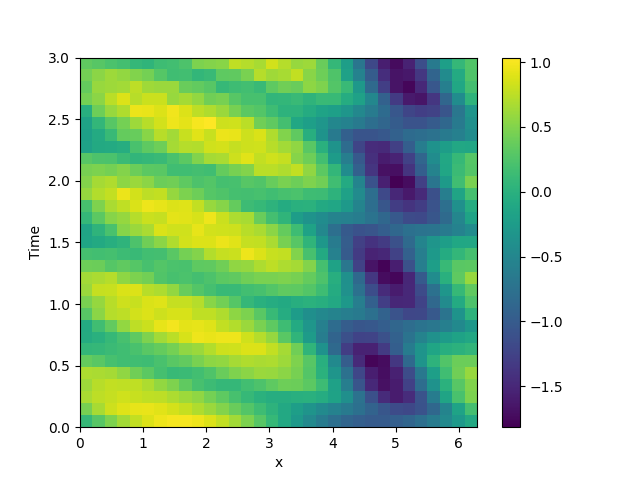# 2.21. 1D problem - Using custom class

This example implements a PDE that is only defined in one dimension. Here, we chose the Korteweg-de Vries equation, given by

$\partial_t \phi = 6 \phi \partial_x \phi - \partial_x^3 \phi$

which we implement using a custom PDE class below.from math import pi

from pde import CartesianGrid, MemoryStorage, PDEBase, ScalarField, plot_kymograph

class KortewegDeVriesPDE(PDEBase):
"""Korteweg-de Vries equation"""

def evolution_rate(self, state, t=0):
"""implement the python version of the evolution equation"""
assert state.grid.dim == 1  # ensure the state is one-dimensional

# initialize the equation and the space
grid = CartesianGrid([[0, 2 * pi]], , periodic=True)
state = ScalarField.from_expression(grid, "sin(x)")

# solve the equation and store the trajectory
storage = MemoryStorage()
eq = KortewegDeVriesPDE()
eq.solve(state, t_range=3, method="scipy", tracker=storage.tracker(0.1))

# plot the trajectory as a space-time plot
plot_kymograph(storage)


Total running time of the script: (0 minutes 3.102 seconds)